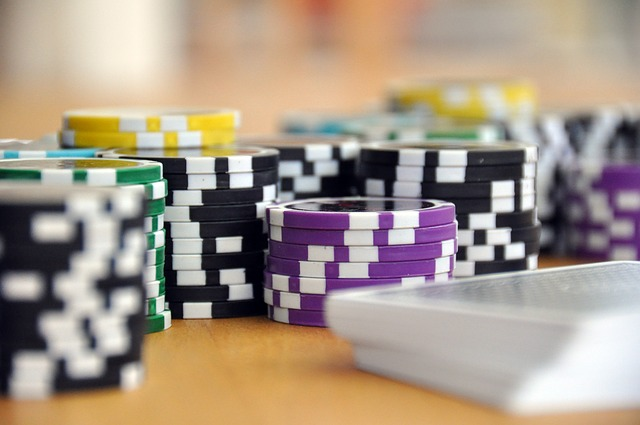October 3, 2023

# Implied Odds are Easier with a Quick Math StrategyImplied odds help casino games players get value out of their bets, but when you play online casino games time limits that dealer’s or croupiers may put you on between bets can send you in to a panic of mathematical calculation.

This is made worse when you have brain freeze, so you end up not being able to do the math and making the wrong call. Thank fully in casino games there are some easy ways to calculate pot odds and implied odds.

Let’s take Ultimate Hold’em Casino Games as An Example

Firstly, you need to understand pot odds. If you look at a pack of cards as 50 cards rather than 52 and divide 100% by 50 you will get 2%, so each card is worth 2%. This means each out is worth 2%. To calculate the percentage of your outs hitting the board by the time the flop cards are on the table, you simply multiply any outs by 2 giving you the percentage of your hand improving.

Secondly, you need to calculate your pot odds. You need to use you percentage of winning versus the amount of money in the pot. This is also quite easy. If there is \$100 in the pot and your opponent has bet \$50, then you need to bet \$50 to try and win \$150. That means you are getting a 3-1 return on your pot odds or 30%. If you have 15 outs then this would be 30.

However, how often do you have 15 outs?

According to an article on c9bets blog section, unless you have a flush and straight draw simultaneously this is not going to happen. As a result you would need to fold most of your hands on the flop. To solve this there is something called implied odds that can help you get past this quick flop fold situation.

Implied odds are basically a prediction of how much you expect the pot to grow by the time the turn card comes out. If your opponent is betting \$50 at \$100 and you call the \$50, the pot is going to be \$200 by the turn. You opponent has a habit of betting 50% of the pot, so you would expect the other player to bet another 50% after the turn, so this would mean you need to take the current pot size and add \$100.

The implied pots odds are now \$250. You want to wager \$50 on the turn for \$250 available on the river giving you a 20% bet and as 20% goes into 100% 5 times your implied pot odds become 5-1. You need 10 outs to justify a call now.

Most people don’t want to use the math above so the easy way to work out the implied pot odds is take the amount of money in the pot already on the flop excluding your opponents bet and double the pot size. This then gives you a rough figure of how much money you expect to be in the pot by the turn and gives you better implied odds to call your opponent.